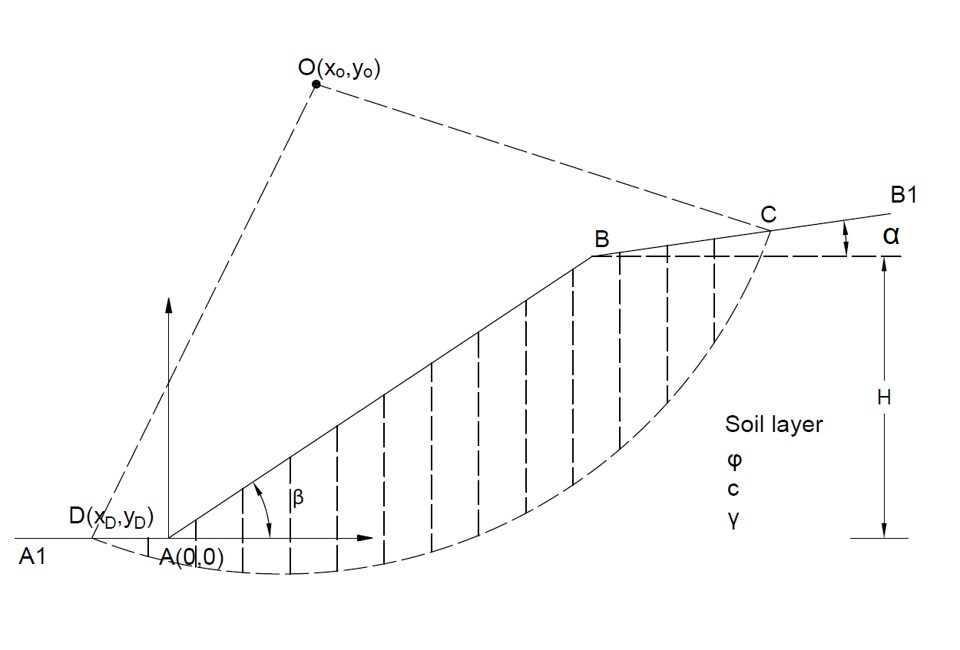Slope Tool (circular sliding)Geometry of Slope Stability (circular sliding)Tips

• This tool is for slope stability analysis, as shown in the figure above.
• The slip surface is assumed as circular.
• The Swedish Method,the Fellenius Method, the Janbu's Method, and Bishop Method are adopted in this tool.
• Before calculation, please choose the applicable unit weight for different conditions.
• The Fellenius Method is only suitable for the condition using saturated unit weight.
• The unit weight of water is 9.8 (kN/m3).
• The start point of slip surface D should be located on AA1 or AB with only xD as input.
• The lower slope angle β should be larger than the upper slope angle α.
• The factor of safety FS is defined as the ratio of resisting moment Mr to driving moment Md.
• If "nan", "0" or "inf" displays in Results, please check your input parameters.

H (m)
β (°)
α (°)
xO (m)
yO (m)
xD (m)
γ (kN/m3)
c (kN/m2)
φ (°)
n

Output Results

• x coordinate of point C
xC (m) =
• y coordinate of point C
yC (m) =